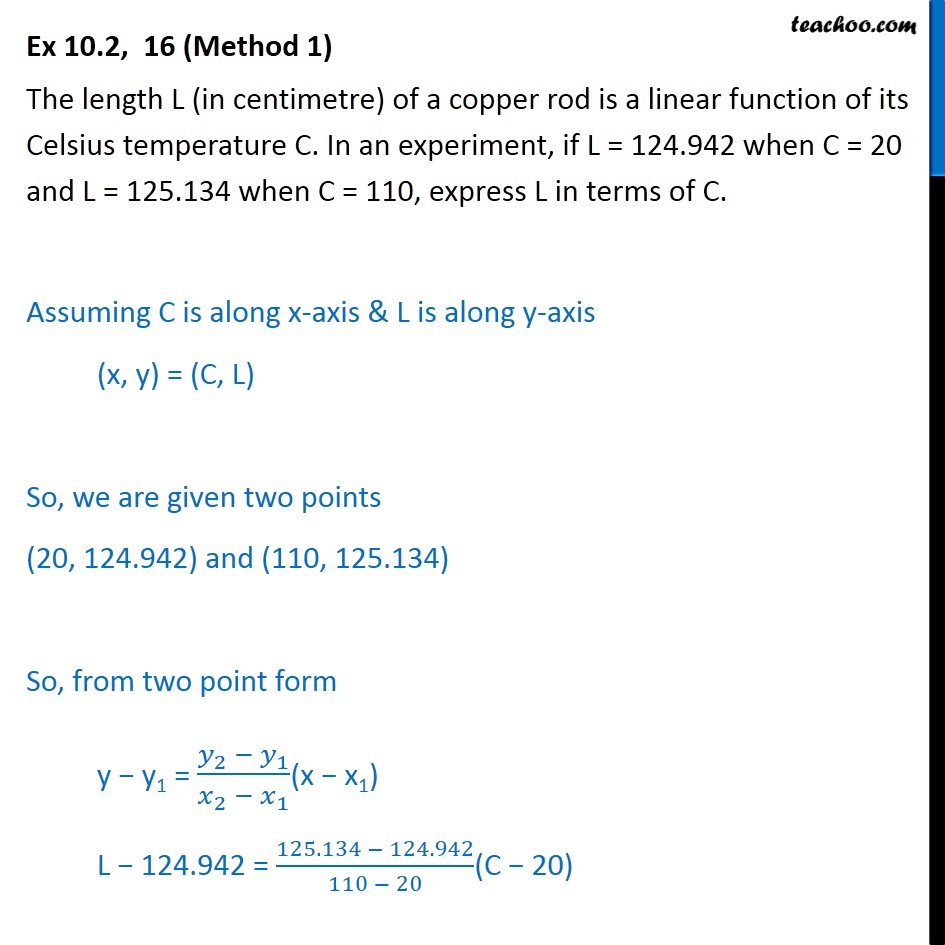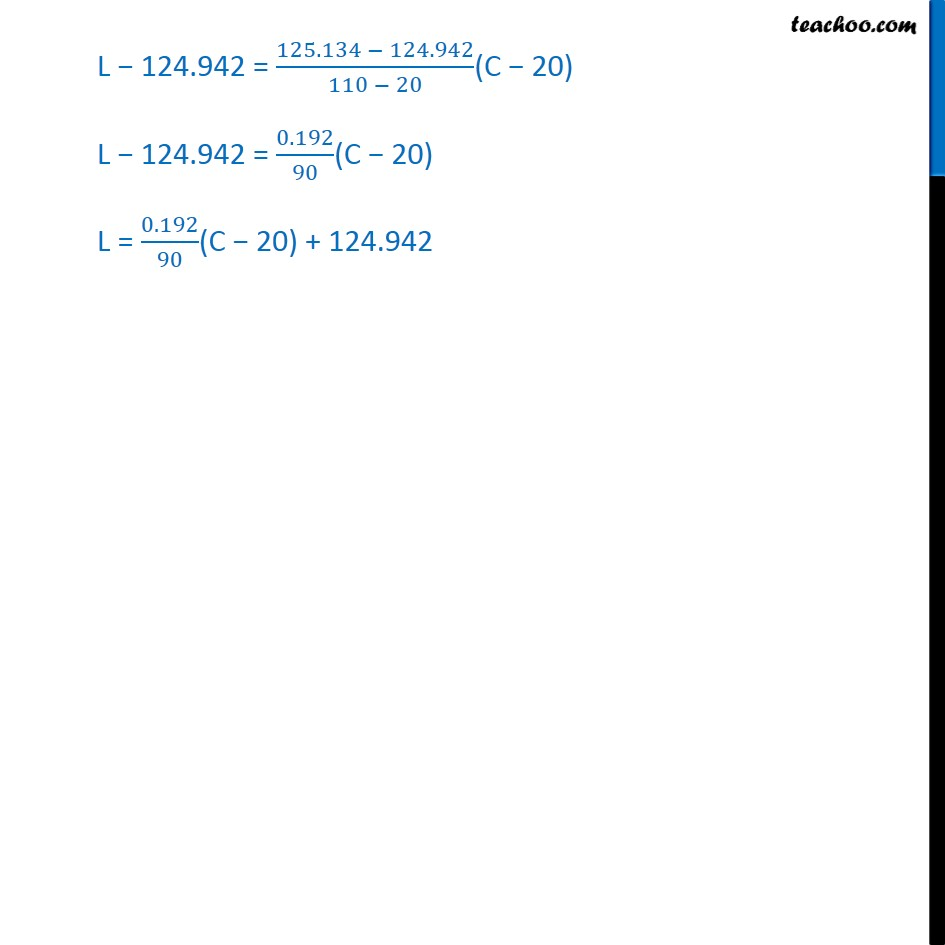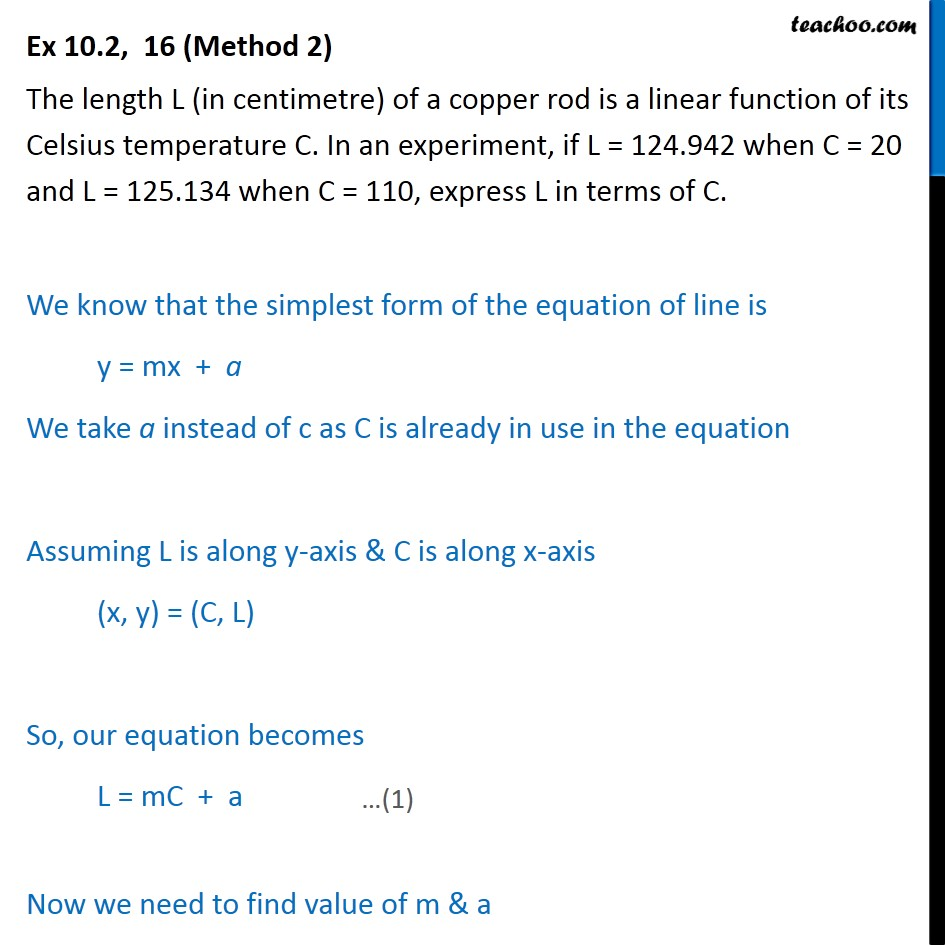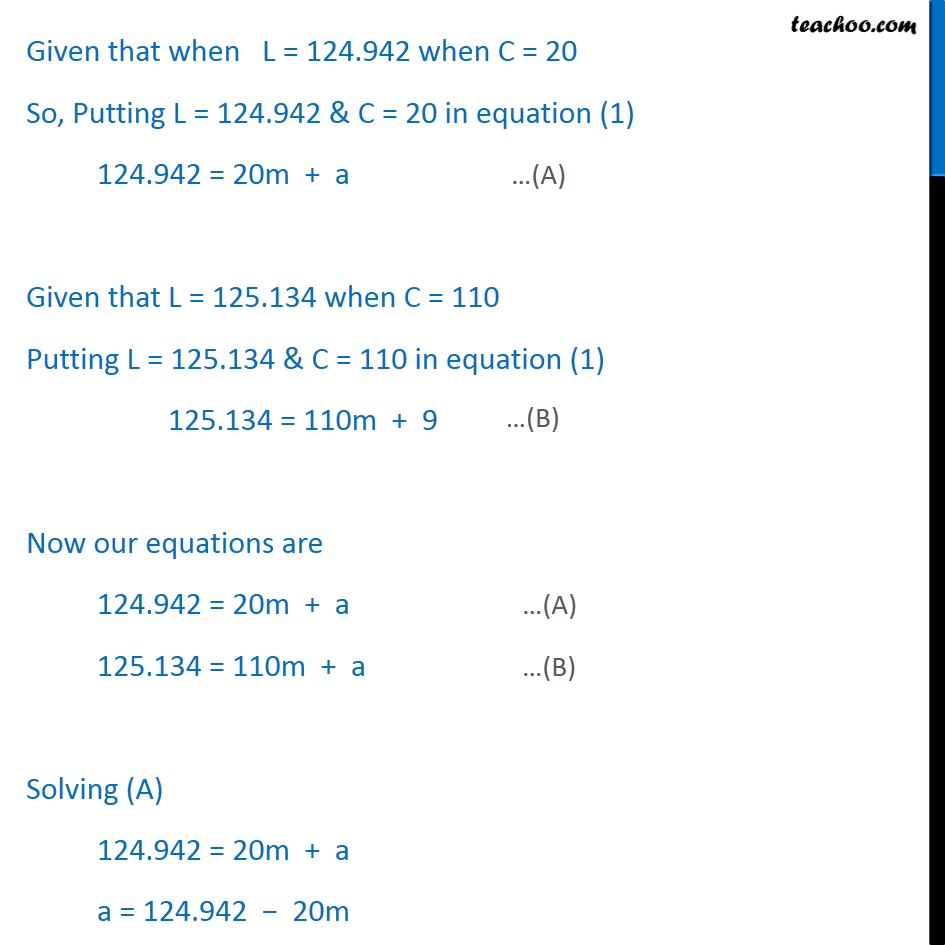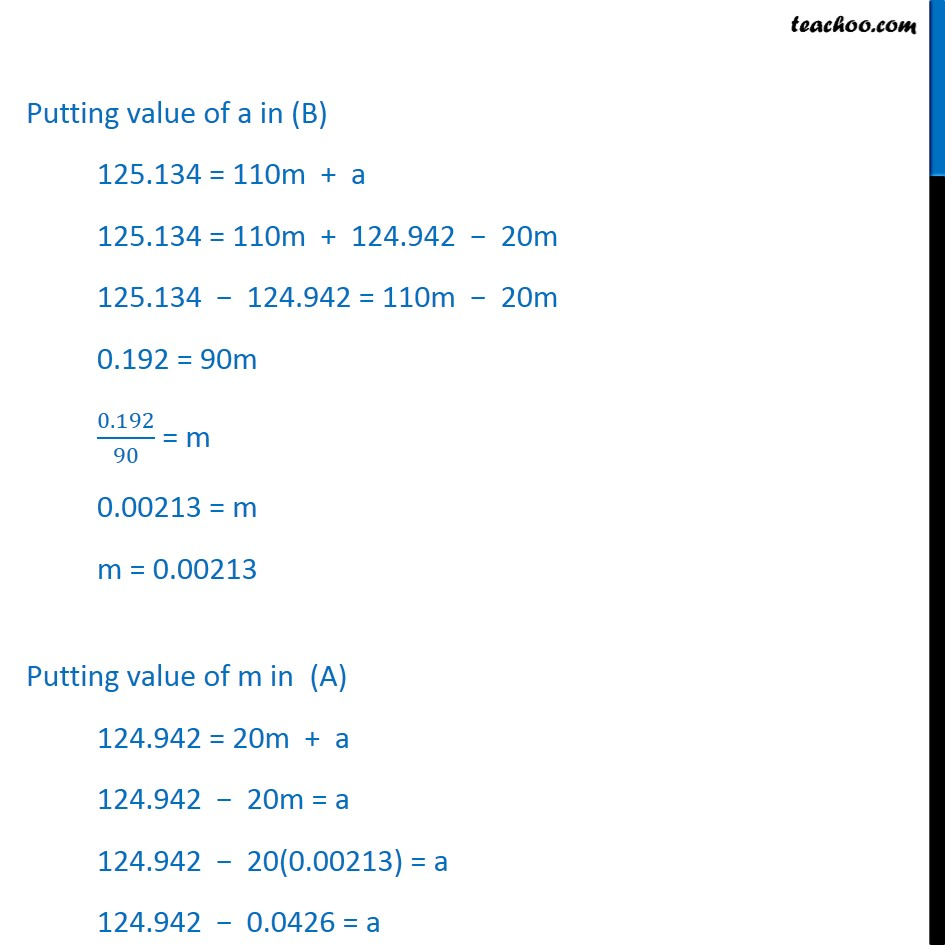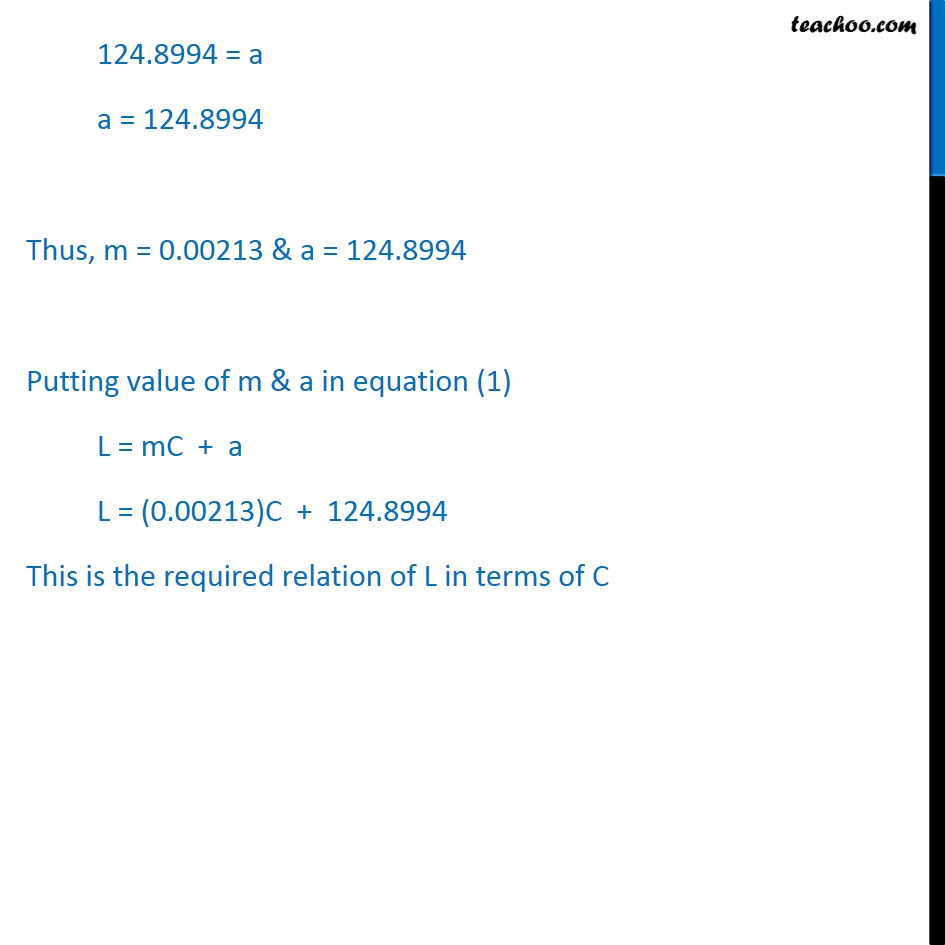1. Chapter 10 Class 11 Straight Lines
2. Serial order wise
3. Ex 10.2

Transcript

Ex 10.2, 16 (Method 1) The length L (in centimetre) of a copper rod is a linear function of its Celsius temperature C. In an experiment, if L = 124.942 when C = 20 and L = 125.134 when C = 110, express L in terms of C. Assuming C is along x-axis & L is along y-axis (x, y) = (C, L) So, we are given two points (20, 124.942) and (110, 125.134) So, from two point form y − y1 = (𝑦_2 − 𝑦_1)/(𝑥_2 − 𝑥_1 )(x − x1) L − 124.942 = (125.134 − 124.942)/(110 − 20)(C − 20) L − 124.942 = (125.134 − 124.942)/(110 − 20)(C − 20) L − 124.942 = 0.192/90(C − 20) L = 0.192/90(C − 20) + 124.942 Ex 10.2, 16 (Method 2) The length L (in centimetre) of a copper rod is a linear function of its Celsius temperature C. In an experiment, if L = 124.942 when C = 20 and L = 125.134 when C = 110, express L in terms of C. We know that the simplest form of the equation of line is y = mx + a We take a instead of c as C is already in use in the equation Assuming L is along y-axis & C is along x-axis (x, y) = (C, L) So, our equation becomes L = mC + a Now we need to find value of m & a Given that when L = 124.942 when C = 20 So, Putting L = 124.942 & C = 20 in equation (1) 124.942 = 20m + a Given that L = 125.134 when C = 110 Putting L = 125.134 & C = 110 in equation (1) 125.134 = 110m + 9 Now our equations are 124.942 = 20m + a …(A) 125.134 = 110m + a …(B) Solving (A) 124.942 = 20m + a a = 124.942 − 20m Putting value of a in (B) 125.134 = 110m + a 125.134 = 110m + 124.942 − 20m 125.134 − 124.942 = 110m − 20m 0.192 = 90m 0.192/90 = m 0.00213 = m m = 0.00213 Putting value of m in (A) 124.942 = 20m + a 124.942 − 20m = a 124.942 − 20(0.00213) = a 124.942 − 0.0426 = a 124.8994 = a a = 124.8994 Thus, m = 0.00213 & a = 124.8994 Putting value of m & a in equation (1) L = mC + a L = (0.00213)C + 124.8994 This is the required relation of L in terms of C

Ex 10.2